# Non inverting amplifier

The non-inverting opamp does not invert the input terminals, so the input voltage is connected to the positive terminal and the negative terminal is connected to ground.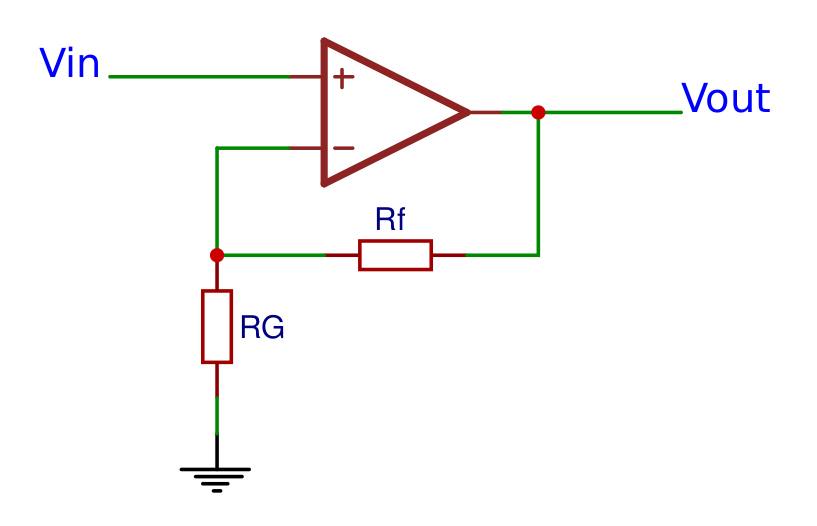### Formulas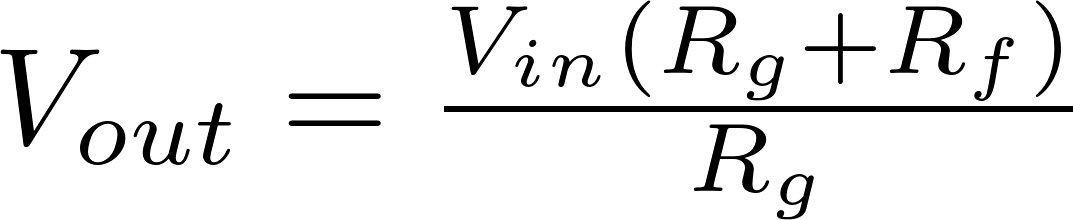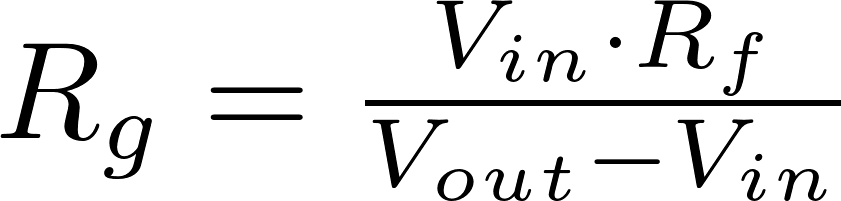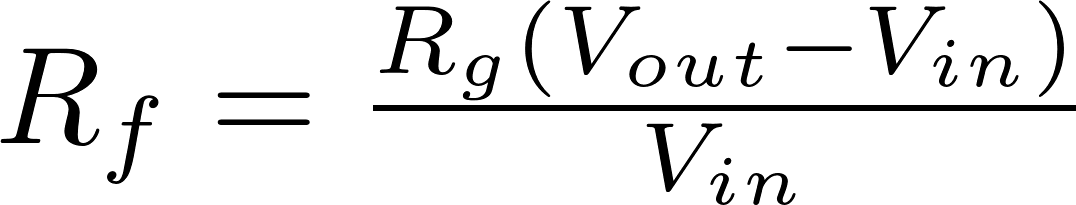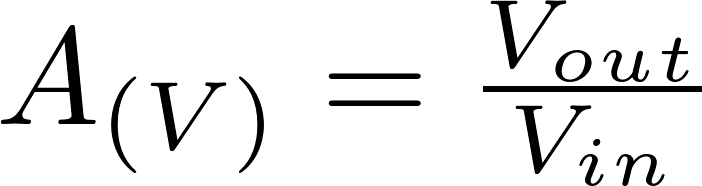V in is the input voltage and is measured in volt (V)
V out is the output voltage and is measured in volt (V)
R is the symbol for resistance and is measured in ohm (Ω).
A is the symbol for gain.